Next: Rotating reference frames Up: Orbits in central force Previous: Perihelion precession of Mercury

# Exercises

1. Derive Equations (5.2) and (5.7).

2. Prove that in the case of a central force varying inversely as the cube of the distance,where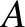,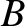,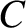are constants. (From Lamb 1923.)

3. The orbit of a particle moving in a central field is a circle that passes through the origin; that is,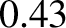, where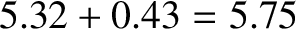. Show that the force law is inverse fifth power. (Modified from Fowles and Cassiday 2005.)

4. The orbit of a particle moving in a central field is the cardoid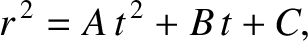, where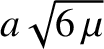. Show that the force law is inverse fourth power.

5. A particle moving in a central field describes a spiral orbit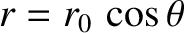, where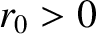,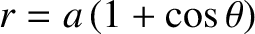. Show that the force law is inverse cube, and that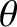varies logarithmically with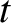. Demonstrate that there are two other possible types of orbit in this force field, and give their equations. (Modified from Fowles and Cassiday 2005.)

6. A particle moves in the spiral orbit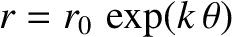, where. Suppose thatincreases linearly with. Is the force acting on the particle central in nature? If not, determine howwould have to vary within order to make the force central. Assuming that the force is central, demonstrate that the particle's potential energy per unit mass is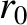where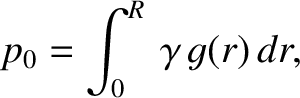is its (constant) angular momentum per unit mass. (Modified from Fowles and Cassiday 2005.)

7. A particle moves under the influence of a central force per unit mass of the form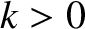where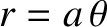and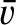are positive constants. Show that the associated orbit can be written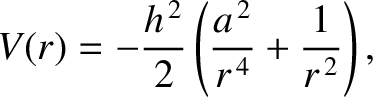which is a closed ellipse for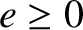and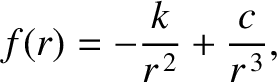. Discuss the character of the orbit for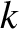and. Demonstrate that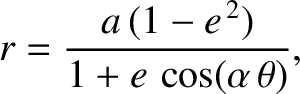where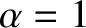.

8. A particle moves in a circular orbit of radiusin an attractive central force field of the form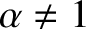, where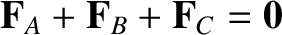and. Demonstrate that the orbit is only stable provided that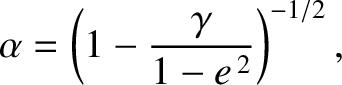.

9. A particle moves in a circular orbit in an attractive central force field of the form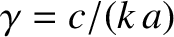, where. Show that the orbit is unstable to small perturbations.

10. A particle moves in a nearly circular orbit of radiusunder the action of the radial force per unit mass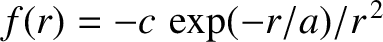where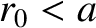and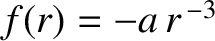. Demonstrate that the so-called apse line, joining successive apse points, rotates in the same direction as the orbital motion through an angle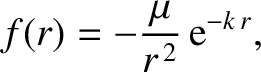each revolution. (From Lamb 1923.)

11. A particle moves in a nearly circular orbit of radiusunder the action of the central potential per unit mass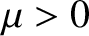whereand. Show that the apse line rotates in the same direction as the orbital motion through an angle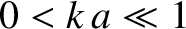each revolution. (From Lamb 1923.)

12. Suppose that the solar system were embedded in a tenuous uniform dust cloud. Demonstrate that the apsidal angle of a planet in a nearly circular orbit around the Sun would be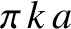where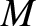is the mass of the Sun, and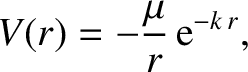is the mass of dust enclosed by a sphere whose radius matches the major radius of the orbit. It is assumed that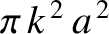.

13. Consider a satellite orbiting around an idealized planet that takes the form of a uniform spheroidal mass distribution of mean radius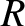and ellipticity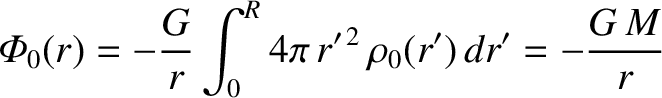(where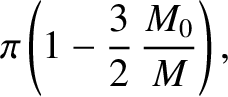). Suppose that the orbit is nearly circular, with a major radius, and lies in the equatorial plane of the planet. The potential energy per unit mass of the satellite is thus (see Chapter 3)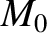where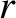is a radial coordinate in the equatorial plane. Demonstrate that the apse line rotates in the same direction as the orbital motion at the rate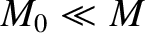where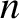is the mean orbital angular velocity of the satellite.Next: Rotating reference frames Up: Orbits in central force Previous: Perihelion precession of Mercury
Richard Fitzpatrick 2016-03-31